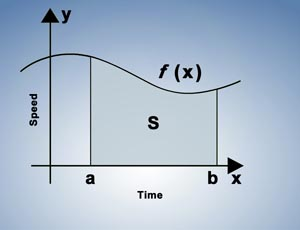StoreSeries • Module: Introduction to CalculusModule: Introduction to CalculusIntroduction to Calculus

Calculus has widespread applications in science, engineering, and economics and can solve many problems for which algebra alone is insufficient. This module introduces the industrial uses of calculus, such as calculating production rate and rate of delivery, and covers topics including derivatives and integrals.

This module takes 30 minutes to complete. At the end of this module, you must obtain an 80% or higher on the Test Your Knowledge for it to be marked as complete. You have unlimited attempts and the highest attempt is recorded.

 \$10.00